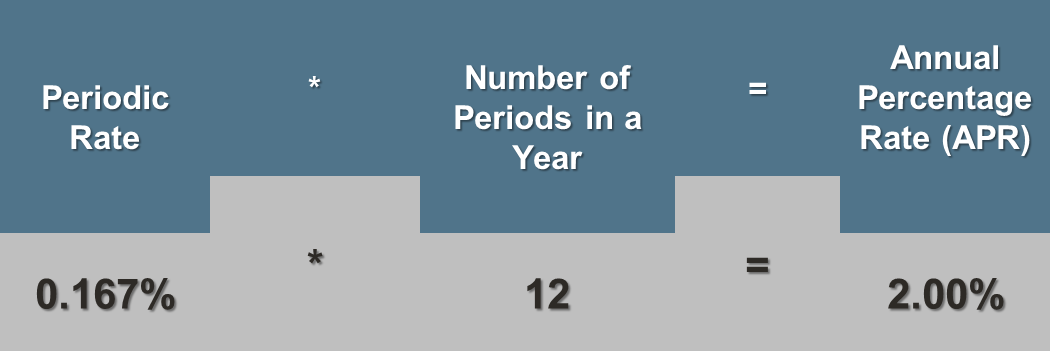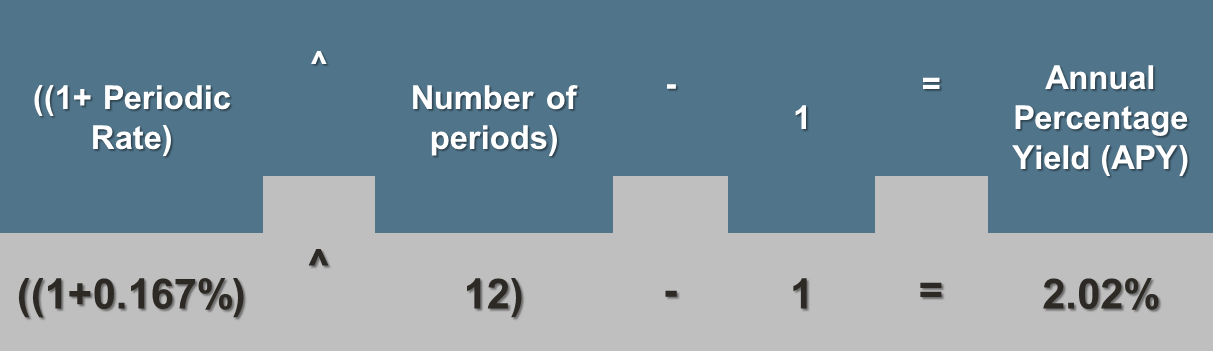<img src="https://events.xg4ken.com/pixel/v2?tid=KT-N2BAB-3ED&amp;noscript=1" width="1" height="1" style="display:none">

When you look at interest rates, you’ll see two abbreviations pop up over and over again—APR and APY. Some financial institutions will show both the APR and APY for their finances, while others may show only one value or the other. To make things more confusing, the rates may only vary by a fraction of a percent.

If you assume APR and APY are pretty much the same thing, you’re not alone. There’s only one letter of difference between the two, after all, and since the numbers are almost the same, it stands to reason that the two terms are almost the same as well.

They’re not, though, and knowing the difference could have a significant effect on how much your investments earn, or how much interest you pay on loans, over time.## Annual Percentage Rate (APR)

The simplest of the two terms is APR, the annual percentage rate. This is the base rate of interest you get on your investment each year, or that you will pay a lender if you have a loan or credit card debt. If you have \$100 in an account with an APR of 2%, for instance, you’ll earn two dollars by the end of the year.

While most financial institutions will tell you the APR up front, it’s actually pretty easy to calculate. First, you need the periodic rate.

The periodic rate is nothing more than how much interest a financial institution charges your loan each period (such as quarterly or monthly). Financial institutions calculate the periodic rate by dividing their APR by the number of periods in a year, so if a bank with an APR of 2% charges your account interest monthly, they’ll simply divide the APR by 12 to get their periodic rate of 0.167%. If the bank charges your account interest quarterly, on the other hand, they will divide 2% by 4 to get a periodic rate of 0.50%.

In order to get the APR, then, all you have to do is multiply the periodic rate by the number of periods in a year. If the periodic rate of interest is 0.167% and charged monthly, for instance, the formula would be:## Annual Percentage Yield (APY)

The APY, or annual percentage yield, on the other hand, is a little more complex. This is because it takes compound interest into account.

If you’ve never heard of compound interest, you’re missing out on what Albert Einstein considered the greatest force on Earth. It’s basically the interest you get paid on the interest you’ve already earned.

Let’s go back a second to our previous example of a savings account with \$100 in it that has a 2% APR. If the interest is distributed monthly at 0.167%, each month your \$100 is getting a little bit of interest, namely 16.7 cents.

That may not seem like much, but the magic happens in the second month, when the bank distributes interest based not on your original \$100, but your new total of \$100.167. Now you’re getting a little bit more back.

Granted, it’s not a lot (\$0.1673 vs. \$0.167, to be exact), but what you need to remember is that each month, you’re getting interest not just on your original \$100, but all the interest you have collected so far. At the end of the year, your total will be \$102.2. That’s more than the 2% annual percentage rate. That value, the APR plus compound interest, is the APY.

You can find out the APY on an investment using this formula, I have included our original example for reference:## How Do APR and APY Affect Me?

When financial institutions talk about their interest rates, they have two different numbers they can use. Since APR is the lower number, many financial institutions will use it when advertising their loans. However, an APR for a loan doesn’t take into consideration the compound interest you’ll have to pay. Instead, you’ll want to look at the APY, which is the rate you’ll actually be paying.

Another example: If you get a home loan with an APR of 9%, you’re not taking into account the periodic rate. If the financial institution charges interest monthly, that puts the APY at 9.38%. That means you’re paying 0.38% more on your loan each month than you thought. That amount can really add up, especially on a 30 year loan where that 0.38% can raise your monthly payments from \$805 at 9% interest to \$832 at 9.38% interest.

Conversely, if you’re looking at savings accounts or CDs, a financial institution will probably advertise their APY interest rate, which is the bigger number. While it will give you an idea of your return on investment, you’ll want to find out what the APR is and the periodic rate. Remember that the more frequently the interest compounds, the bigger the difference between the APR and the APY.

Knowing what APR and APY represent can make a big difference in your personal finances. Not only will you have a clearer idea of the deal a financial institution is offering you, you’ll also be better able to confidently compare rates from different financial institutions.